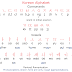## Recent Posts# Korean Alphabet: How to Read, Write, and Pronounce

## Lesson 1: Pronounce the Korean Alphabet

The 14 basic Korean characters:
1. 가 (gah) = It may sound like a K, but there is another Korean consonant that has a stronger K sound (ㅋ). Think of ㄱ as a soft G sound.

2. 나 (nah) = it's easy, right?

3. 다 (dah) = Might sound like “tah,” however the ㅌ consonant has a stronger T pronunciation. Think of ㄷ as a soft D.

4. 라 (lah) = The ㄹ consonant has a rolling L sound. It may sound like an R, however Koreans do not have a consonant that is a 100% R equivalent. Thus if a native Korean says, “Rock and roll,” it’ll sound more like “Lock and Loll.”

5. 마 (mah) = Sounds like the second “ma” in “mama.”

6. 바 (bah) = It may sound like a P, but there is another Korean consonant with a stronger P sound (ㅍ). Think of ㅂ as a soft B sound.

7. 사 (sah) = Did you know 사 means the number “four”?
8. 아 (ah) = The ㅇ consonant, which looks like a circle, is silent. However when paired with other characters, the ㅇ consonant can take the sound of a previous consonant.
• 아 (ah)
• 야 (yah)
• 어 (uh)
• 여 (yuh)
• 오 (oh)
• 요 (yoh)
• 우 (ooh)
• 유 (you)
• 으 (euh)
• 이 (e)
9. 자 (jah) = Might sound like “cha,” think of ㅈ as a soft J sound. However the ㅊ Korean consonant has a stronger “ch” pronunciation.

10. 차 (cha) = Pronounced the same as the Cuban dance “Cha Cha.”

11. 카 (kah) = It's easy.

12. 타 (tah) = A simple one!

13. 파 (pah) = 파 means “spring onion.”

14. 하 (hah) = Pronounced the same as the “ha” when someone laughs “hahaha.”

## Lesson 2: Write the Alphabet (Basic Consonants + Vowels)

Download worksheet for practicing writing basic consonants and vowels: worksheet 1 | worksheet 2